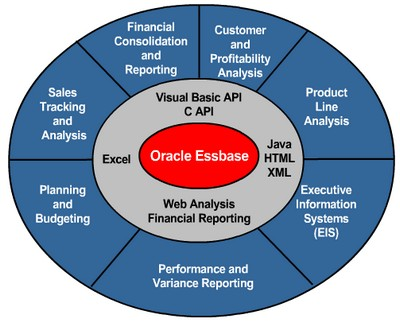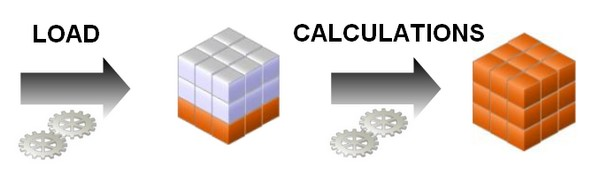# Essbase - Variance Reporting

Variance reporting properties determine how Essbase calculates the difference between actual and budget data in a member with the @VAR or @VARPER function in its member formula. Any member that represents an expense to the company requires an expense property.

When you are budgeting expenses for a time period, the actual expenses should be less than the budget. When actual expenses are greater than budget expenses, the variance is negative. The @VAR function calculates Budget – Actual. For example, if budgeted expenses are 100, and you spend 110, the variance is -10.

When you are budgeting nonexpense items, such as sales, the actual sales should be more than the budget. When actual sales are less than budget, the variance is negative. The @VAR function calculates Actual – Budget. For example, if budgeted sales were 100, and you made 110 in sales, the variance is 10.

By default, members are nonexpense.

The variance reporting calculation requires that any item that represents an expense to the company must have an expense reporting tag.

## Variance reporting properties

Essbase provides two variance reporting properties:

• expense
• and nonexpense.

The default is nonexpense.

This tag are available exclusively for use with Essbase - (Accounts|Measures) Dimensions members.

## @VAR or @VARPER function

Variance reporting properties define how Essbase calculates the difference between actual and budget data in members with the @VAR or @VARPER function in their member formulas.

When you tag a member as expense, the @VAR function calculates Budget – Actual. For example, if the budgeted amount is 100 and the actual amount is 110, the variance is –10.

Without the expense reporting tag, the @VAR function calculates Actual – Budget. For example, if the budgeted amount is 100 and the actual amount is 110, the variance is 10.

## Documentation / Reference

Discover MoreEssbase - (Accounts|Measures) Dimensions

Accounts dimensions are dimension that contains items that you want tomeasure, and makes Essbase built-in accounting functionality available. Accounting classifications. Ex: income expense profit...Essbase - Calculations

Essbase includes powerful calculation features for demanding analytic requirements. A rich library of functions makes it easy to define advanced and sophisticated business logic and relationships. Essbase...Essbase - The OLAP Design Cycle (to create an optimized database)

A contains two types of values : the values that you enter or load (input data) and the values that are calculated from the input data (calculated data) Then a consolidatDesigning and Building...